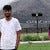# Bit-manipulation: a useful topic for Competitive Programming

Hello there ✋, Today I am going to tell you some useful concepts of bitwise operators which you can use in competitive programming to improve the efficiency of your code.

First Let us Understand all Bitwise Operators one by one.

## 1. BITWISE AND (&) Operator

This bitwise & operator takes two bits/numbers as operands and return 1 if both bits are 1 otherwise return 0.

Ex: 1&1 = 1, 1&0 = 0, 0&0 = 0.

## 2. BITWISE OR(|) Operator

This bitwise | operator takes two bits or numbers as operands and return 0 if both bits are 0 otherwise return 1.

Ex: 1|1 = 1, 1|0 = 1, 0|0…

# Sudoku — A Logical Puzzle

Hello there! we all have heard about a very famous logical puzzle called “Sudoku”. Today i am going to tell you about this puzzle, one of the way for solving this puzzle and on which platform you can solve it. So let’s start.

So Basically Sudoku is a logical puzzle that is represented by a 9x9 Grid, and we have to fill a number range from 1 to 9 in each and every cell, but there is some rule for filling the numbers.

1. Fill the number in a cell such that no other cell contains that number in the same…

# The Magic of In-place Algorithm

Hey there! Today i am going to publish my first article on In-Place Algorithm . Basically I came to know about this algorithm while solving a question on leetcode. I found this algorithm very useful as it uses constant space for solving a question, However it uses some variables for computation which are of constant space.

## What does In-place Algorithm do?

Basically What happens? Whenever we give input to this algorithm, it takes that input, perform some computation on input itself, use some extra varaible (according to requirement). So what we get as a output? …## Swapnil agrawal

A passionate Computer programmer, making my path towars development field.